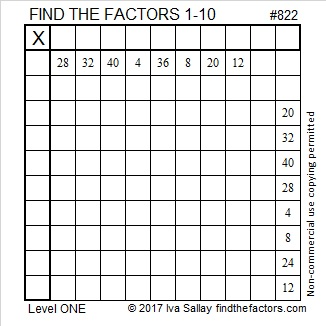# 822 and Level 1

The sum of 822’s digits is 12, a number divisible by 3. That means that even number 822 can be evenly divided by 2, 3, and 6.

822 is the sum of the 12 prime numbers from 43 to 97.

822 is palindrome 212 in base 20 because 2(20²) + 1(20¹) + 2(20º) = 822.Print the puzzles or type the solution on this excel file: 10-factors-822-828

• 822 is a composite number.
• Prime factorization: 822 = 2 x 3 x 137
• The exponents in the prime factorization are 1, 1, and 1. Adding one to each and multiplying we get (1 + 1)(1 + 1)(1 + 1) = 2 x 2 x 2 = 8. Therefore 822 has exactly 8 factors.
• Factors of 822: 1, 2, 3, 6, 137, 274, 411, 822
• Factor pairs: 822 = 1 x 822, 2 x 411, 3 x 274, or 6 x 137
• 822 has no square factors that allow its square root to be simplified. √822 ≈ 28.67054